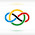### 2003 IMO SL #C1

Let $A$ be a $101$-element subset of the set $S=\{1,2,\ldots,10^6\}$. Prove that there exist numbers $t_1$, $t_2, \ldots, t_{100}$ in $S$ such that the sets$A_j=\{x+t_j\mid x\in A\},\qquad j=1,2,\ldots,100$are pairwise disjoint.

Consider the set $$D=\{x-y \mid x,y \in A\}.$$Note that there are at most $101 \cdot 100 + 1 = 10101$ elements in $D$, where the $1$ accounts for $x=y$. Also, notice that $$A_i \cap A_j = \emptyset \iff (t_i - t_j) \notin D \qquad (\star).$$Therefore, we need to pick the $100$ elements such that we don't use a difference from $D$. Now choose these elements using simple induction. Suppose $k \leq 99$ elements are already chosen. Also, due to $(\star)$, note that if an element $x$ is selected, we cannot choose any element from the set $x + D$. Hence, after $k$ elements are chosen, we cannot include at most $10101k \leq 999999$ elements. But since $999999=10^6-1$, we can select one more element, as desired. $\square$

1.Anonymous1/09/2022

o
nice solution

2.very clean!

3.Olivia1/11/2022

How did you get that there are 101⋅100+1 elements in D?

4.Thanks @Anonymous and @EZmath :)

@Olivia
There are two cases, x=y and x≠y. If x=y, then no matter what x,y are, x-y will always correspond to the element 0. If x≠y, then assume x>y. Because there are 101 elements in A, there are 101 ways to choose x. Then, we have 100 elements remaining to choose from in A, so there are a 100 ways for y. It follows that the total number of elements in D is 101⋅100+1.